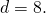Midterm 1 Preparation

# Midterm 1: Version D

1. Evaluate: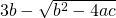if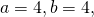and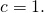2. Solve for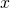in the equation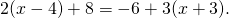3. Isolate the variable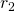in the equation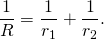4. Solve forin the equation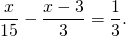5. Write the equation of the horizontal line that passes through the point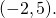6. Find the equation that has a slope of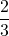and passes through the point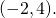7. Find the equation of the line passing through the points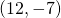and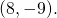8. Graph the relation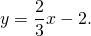For questions 9 to 11, find each solution set and graph it.

1.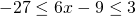2.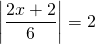3.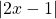>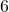4. Graph the relation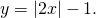5. For a given triangle, the first and second angles are equal, but the third angle is 10° less than twice the first angle. What are the measures of the three angles?
6. Find two consecutive even integers such that their sum is 20 less than the first integer.
7.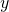varies jointly with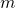and the square of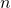and inversely with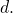If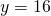when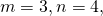and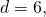find the constant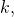then use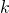to findwhen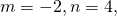and